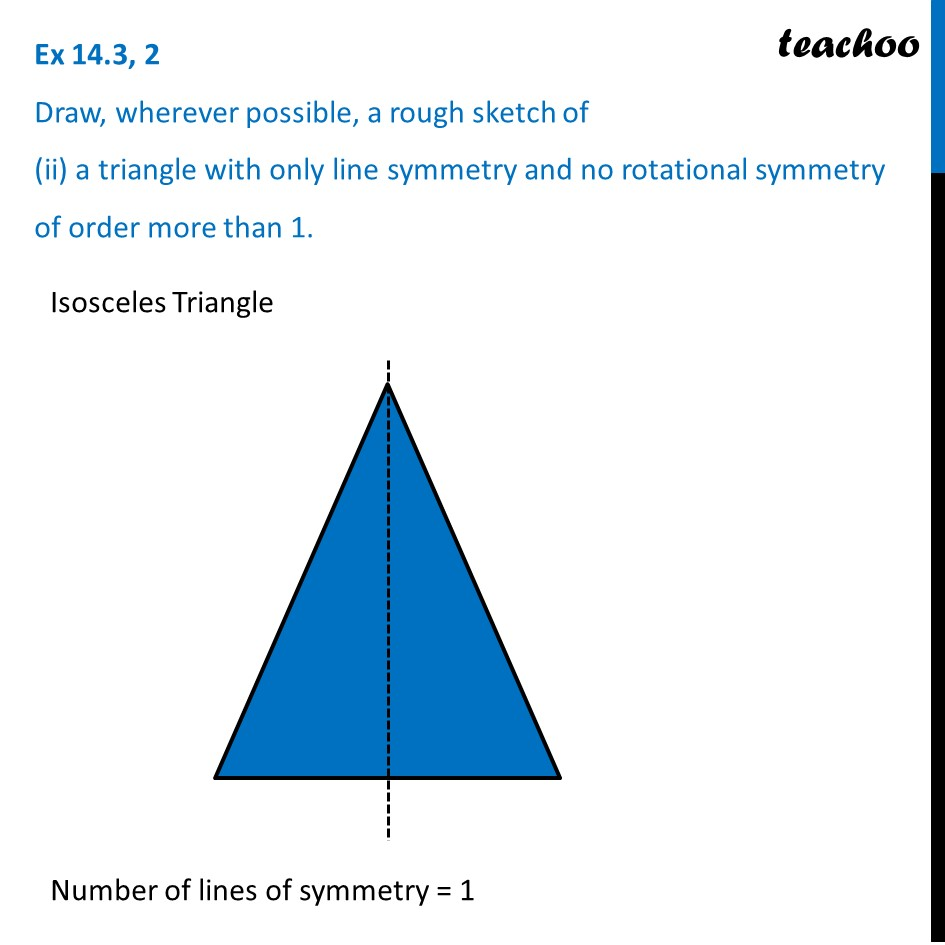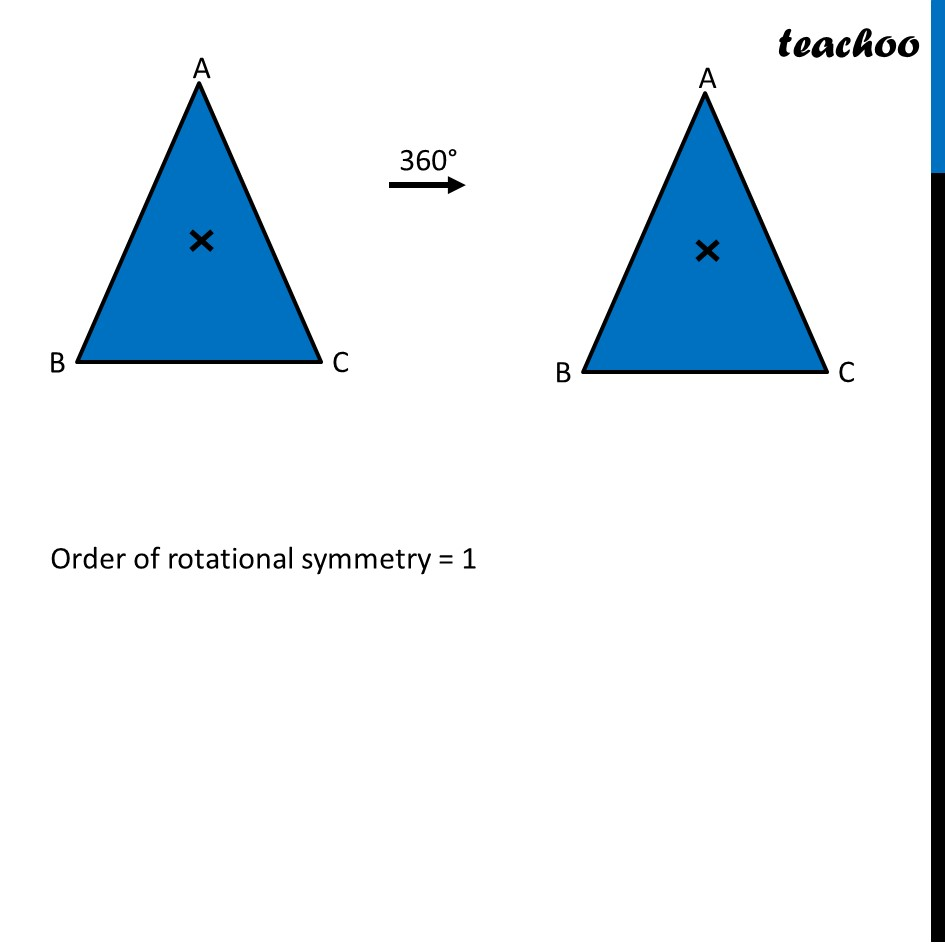1. Chapter 14 Class 7 Symmetry
2. Serial order wise
3. Ex 14.3

Transcript

Ex 14.3, 2 Draw, wherever possible, a rough sketch of (ii) a triangle with only line symmetry and no rotational symmetry of order more than 1.Isosceles Triangle Number of lines of symmetry = 1 Order of rotational symmetry = 1

Ex 14.3

Chapter 14 Class 7 Symmetry
Serial order wise